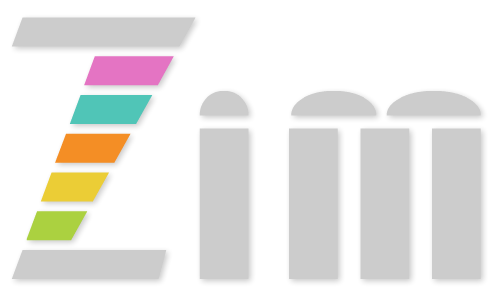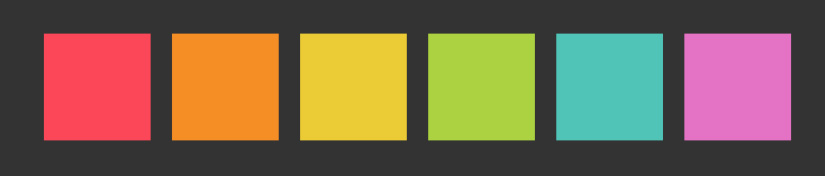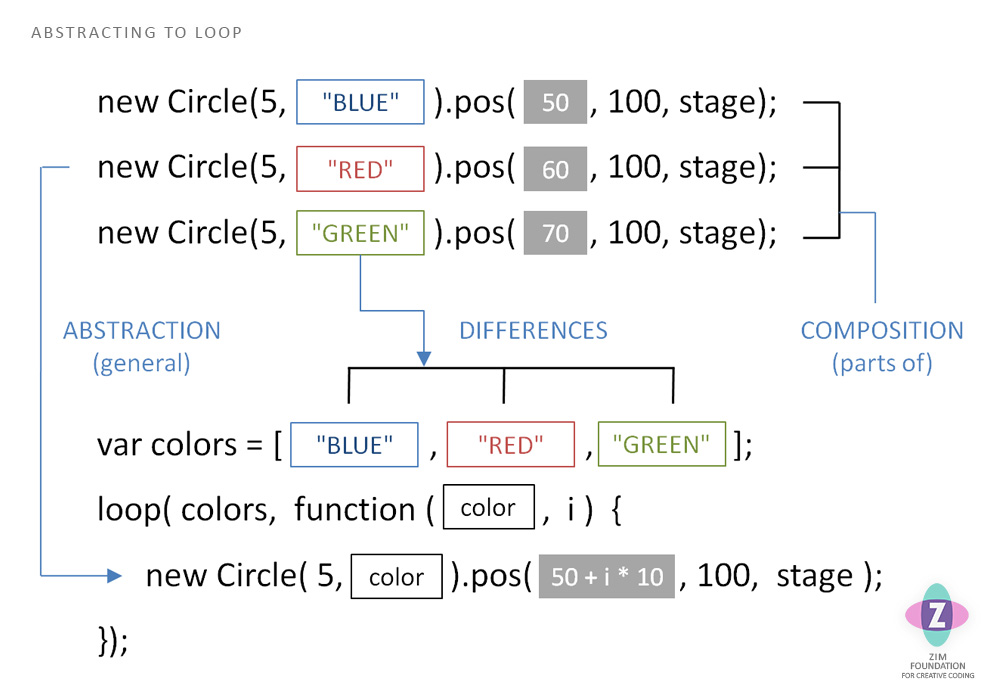# ZIM - Code and Learn Coding with ZIM for JavaScript and HTML Canvas with CreateJS## Practice with ZIM CODE see the Learn Page for tutorials

 ALL LESSONS# Lesson 05 - Arrays and Loops

Creative Coding Videos are above at right - they are a few versions behind the current ZIM.
Differences are that time is now in seconds not milliseconds and ES6 is now used not ES5.

## Theory

In the last lesson we looked at abstraction and how it leads to classification. Classification is very important pattern in OOP object oriented programming - as it is with organization and creativity in life.

Another important pattern is composition. Composition is the parts that things are made from. Each line in our code is composition. The visual parts of our app are composed of objects made from classes - like nouns in the real world. We compose these on the stage and in containers.Often, in composition, we have common code - for instance, tiling six squares of different colors. We can use a loop to run the common code in one place. Unfortunately, each square is a different color and in a different x position. So we abstract the colors and place them in an array. An array is a data structure that holds a list of objects. Then we can loop through the array and each time make a square that matches the color in the array. We can use the loop number (index) to change the position of the square.

Let's take a look at REFERENCE and PRACTICE sections for arrays and loops!

## Reference

Arrays ► What IZ an Array?
An array is a data object that is a list of objects. In JavaScript, we can hold any type of objects in an array. Usually, it is best to store the same type of object in an array. For instance, a list of Numbers, or a list of Strings, etc. We call each object an element of the array and they each have an index number that starts at 0.

01
```// DEFINING AN ARRAY
// we can make a new Array in the traditional way:
const verbs = new Array("wonder", "jump", "point");

// but we tend to use the Array Literal []:
const verbs = ["wonder", "jump", "point"];

// use the array access operator []
// to access the elements of the array
// inside the [] put an index number
// starting at 0 for the first element
zog(verbs); // "wonder"

// CHANGING AN ARRAY
// again, use the array access operator []
verbs = "shrug"; // replace "point" with "shrug"
```

02
```// ARRAY PROPERTIES
// Arrays have a single property:
zog(verbs.length); // logs 3 to the console

// ARRAY METHODS
// There are many array methods
// Here we add and remove elements
verbs.push("call"); // adds to end of array
verbs.pop(); // removes from end of array (and returns value)
verbs.shift(); // removes from start of array (and returns value)
verbs.unshift("smile"); // adds to start of array

verbs.sort(); // sorts array by alphabet **
verbs.indexOf("shrug"); // returns index of element (2) or -1 if not found
verbs.splice(2, 0, "shout"); // inserts shout at index 2 replacing 0 elements
verbs.concat(["paint", "skip"]); // adds second array to verbs
// etc.

** ZIM provides a shuffle(array) function that shuffles the array
```

03
```// MULTIDIMENSIONAL ARRAYS
// Arrays can hold arrays which can hold arrays, etc.
const rounds = [["a", "b", "c"], ["d", "e", "f"]];

// we then use multiple array access opertators to access inside:
zog(rounds); // logs "f" to the console
```

Objects or Arrays?
Both objects and arrays hold data - so which one do we use? The simple answer is use objects for classification and arrays for composition.

04
```// OBJECTS AND ARRAYS
// note that the object holds different properties
// whereas the array holds the same type of values

{color:"red", size:20, dashed:false} // classification
["red", "green", "blue"] // composition

// CLASSIFICATION
// the object holds properties
// belonging to a class of things with these properties
// an object is single thing
// created from this class or that class or that class, etc.
// ors are classification

// COMPOSITION
// the array holds values that can be applied to a set of objects
// such as the colors of three rectangles
// rectangle 1 and rectangle 2 and rectangle 3
// ands are composition

// OPPOSITE
// we can force objects and arrays to hold the opposites
// but it is not very clear in one case or efficient in the other:
["red", 20, false] // we don't know what these things are
{color1:"red", color2:"green", color3:"blue"} // ugg
```

Let's practice Arrays and then we will look at loops and using loops and arrays for abstraction.

## Practice

Arrays are pretty easy. They are just a list of things separated by commas (,). We put them in [] and then to access any element we use the array name[index].

1 Arrays Show

## Reference

Loops ► What IZ a Loop? ► What IZ a ZIM Loop?
Loops let us repeat a block of code. This is a nice efficiency so we can make 100 monsters for instance! We will look at for loops, while loops and ZIM loop().

05
```// FOR LOOP
// this is the most common loop (unless coding in ZIM)
// use let for the variable i as the value changes
// we often use i for iterator - but other variable names are fine too
// (initial condition; condition to keep looping; what to do each loop)
for (let i=0; i<10; i++) {
zog(i); // logs 0-9 on new lines
}

// FORMAT
// note the similar format:
// for () {}
// function () {}
// if () {}

// LOOP THROUGH ARRAY
const colors = ["red", "green", "blue"];
for (let i=0; i<colors.length; i++) {
zog(colors[i]); // logs "red", "green", "blue" on new lines
}

// CONTINUE AND BREAK
for (let i=0; i<10; i++) {
if (i == 2) { // we will see conditionals next lesson
continue; // go to next loop
}
if (i > 5) {
break; // end the looping
}
zog(i); // logs 0, 1, 3, 4, 5
}
```

06
```// WHILE LOOP
let rand = rand(); // rand changes so use let not const
while (rand > .5) {
zog(rand); // consecutive numbers bigger than .5
rand = rand();
}

// and a for loop recreated:
let i = 0;
while (i<10) {
zog(i); log 0-9 on new lines
i++;
}
```

07
```// ZIM LOOP
// a very handy format for looping in a variety of situations
// would recommend using this when coding in ZIM

// NUMBER
loop(10, i=>{
zog(i); // logs 0-9 on new lines
});

loop(10, (i,t)=>{ // second parameter (after i) is total
zog(i, t); // logs 9-0 (reverse) and total 10 each line
}, true); // true reverses loop - see docs for more parameters

// ARRAY
const colors = ["red", "green", "blue"];
loop(colors, color=>{ // second parameter is i, third is total
zog(color); // logs "red", "green", "blue" on new lines
});

// OBJECT
const site = {url:"https://danzen.com", name:"Dan Zen"};
loop(site, (property, value)=>{ // third parameter is i, fourth is total
zog (property + " has " + value); // the properties and values on new lines
});

// CONTAINER
// imagine a container with 100 circles
loop(circles, circle=>{ // second parameter is i, third is total
if (circle.hitTestPoint(100, 100)) { // conditionals next lesson
circle.removeFrom(circles);
stage.update();
}
}, false); // always loop backwards when removing objects from containers

// HOW TO CONTINUE AND BREAK
if (i == 2) { // we will see conditionals next lesson
return; // simple return goes to next loop (instead of continue)
}
if (i > 5) {
return i; // return value to end looping (instead of break)
}
zog(i); // logs 0, 1, 3, 4, 5
});
// collecting the return value is optional - but sometimes handy

// FORMAT
// note the similar format:
loop(10, i=>{});
on("click", e=>{});
timeout(1000, obj=>{});
interval(1000, obj=>{});
```

Abstraction
If we see a bunch of code being repeated over and over, we can abstract the code and place it in a loop. Anything that is different we can place in an array. Often, there is a numerical difference - perhaps we have tiling and each object is moved over. In that case we may just be able to use a calculation with the index number.Let's try out loops in a PRACTICE section!

## Practice

Loops provide great efficiency as we are about to see. Anytime you have repetitive code, consider adding the code once in a loop.

2 For Loop Show
3 ZIM Loop Show
4 Abstraction Show

## Summary

An Array is a way to store a bunch of objects in a list. Usually, these objects are related (the same type of thing). This is easier than storing a variable for each one. Also, a loop helps us repeat code and works really nicely with arrays because we can loop through the array using the index number (starting at 0).

JavaScript uses a for loop. ZIM has wrapped the for loop in a slightly easier function called loop(). This allows us to loop through various data formats such as number, array, object, and container. A regular for loop can do this too - but it takes a few extra steps.

 VIDEOS ALL LESSONS